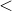# 11.22 for

```for <variable> = <start> to <end> [step <step>]
[loopname <loopname>]
<code>

for (<initialise>; <criterion>; <step>)
<code>
```

The for command executes a set of commands repeatedly. Pyxplot allows for loops to follow either the syntax of the BASIC programming language, or the C syntax.

In the BASIC variant, a specified variable takes a different value on each iteration. The variable takes the value start on the first iteration, and increases by a fixed value step on each iteration; step may be negative if endstart. If step is not specified then a value of unity is assumed. The loop terminates when the variable exceeds end. The following example prints the squares of the first five natural numbers:

```for i = 1 to 5
{
print i**2
}
```

In the C variant, three expressions are provided, which are evaluated (a) when the loop initialises, (b) as a boolean test of whether the loop should continue iterating, and (c) on each loop to increment/decrement variables as required. For example:

```for (i=1,j=1; i<=256; i*=2,j++) { print "%3d %3d"%(j,i); }
```